# 5. selection — Model selection and validation¶

## 5.1. Information Criteria¶

In maximum likelihood methods, the Bayesian and Akaike information criteria give a measurement

Let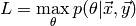be the likelihood of the most probable model parameters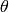with respect to the training data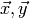. Furthermore, let the model have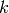free parameters that are estimated and let there be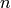training samples. The two information criteria are defined in the following way:

1. Bayesian information criterion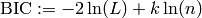2. Akaike information criterion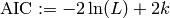From this definition, it can be seen that the criteria add a complexity penalty to the parameter likelihood. The reason behind this is the following: it’s assumed that the higher the number of parameters, the better any set of training data can be approximated by the model. For example, consider polynomial fitting. The number of free parameters corresponds to the order of the fitted polynomial. Given a degree equal to the number of training samples, the polynomial can be fitted perfectly. But as it will be a curve through all given data pairs, the generalization power will be poor. The goal is to find a model with reasonable number of parameters but at the same time a low training error (i.e. a high maximum likelihood).

If the error is normal i.i.d with variance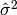, the criteria can be stated as: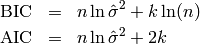Instead of the Akaike information criterion, one should rather empoly the corrected AIC: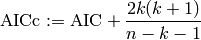Asgets large, the two criteria are identical. Ifis relatively small, the original AIC may suggest models with a larger number of parameters, thus is more likely to be subject of overfitting.

## 5.2. K-Fold Cross-Validation¶

The cross-validation method tries to estimate the generalization error of a model, given some training data. For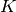-fold cross-validation, the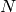samples are split intosubsets of equal size. Then,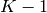subsets are used as training set and the remaining subset as testing set:

1. Split the data intosubsets.

2. For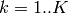1. Fit the model using all subsets except the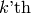.
2. Compute the testing error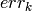on thesubset.
3. The estimated generalization error is the average of the errors of thetesting sets.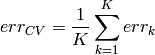If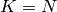, each sample is once used as testing set (consisting of only this sample). This special case is called Leave-one-out cross-validation.

## 5.3. Random Sample Consensus¶

Given a set of training data that is noisy and contains outliers, i.e. erroneous data. The aim of the RANSAC algorithm is to determine which data points are outliers and compute the model without those.

Given the minimal number of points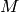required to fit the model and a threshold, the algorithm does the following:

1. Iterate until convergence

1. Out of all data points, selectpoints randomly (the root set).
2. Fit the model to these.
3. Compute the prediction error for all data points.
4. Add all points for which the error is below a threshold to the consensus set of this iteration.
2. Fit the model using all points in the largest consensus set.

As convergence criterion, the probability that at least one outlier free root set was chosen can be tracked. Let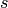be the number of iterations and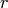the ratio of inliers and outliers.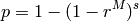Becauseis not known beforehand, it is estimated by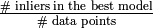. The algorithm iterates, until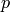exceeds some value (usually close to one, e.g.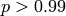).

## 5.4. Interfaces¶

ailib.selection.crossValidation(data, model, K)
Parameters: data (STD) – Data points. model (ailib.fitting.Model) – Model to fit the data points to. K (int) – Number of buckets. Cross-validation error.
class ailib.selection.Ransac(thres, minPts, prob=0.98999999999999999, maxIter=100)
Parameters: thres (float) – Error threshold. prob (float) – Minimum probability of finding an outlier-free root set. maxIter (int) – Maximum number of iterations. minPts (int) – Minimum number of points the model requires to be computed.
fit(data, model)
Parameters: data (STD) – Training data. model (ailib.fitting.Model) – Model to be fit. model instance, fitted to the optimal consensus set.